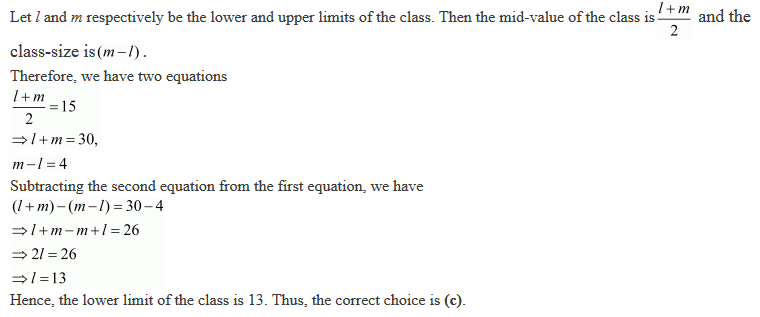#### Chapter 22 Tabular Representation of Statistical Data R.D. Sharma Solutions for Class 9th MCQ's

Mark the correct alternative in each of the following:

1. Tally marks are used to find
(a) Class intervals
(b) Range
(c) Frequency
(d) Upper limits

Solution

Tally marks are used to find the frequencies.
Hence, the correct choice is (c).

2. The difference between the highest and lowest values of the observations is called
(a) Frequency
(b) Mean
(c) Range
(d) Class-intervals

Solution

The difference between the highest and lowest values of the observations is called the range. Hence, the correct choice is (c).

3. The difference between the upper and the lower class limits is called
(a) Mid-points
(b) Class size
(c) Frequency
(d) Mean

Solution

The difference between the upper and the lower class limits is called the class size. Hence, the correct choice is (b).

4. In the class intervals 10-20, 20-30, 20 is taken in
(a) The interval 10-20
(b) The interval 20-30
(c) both intervals 10-20, 20-30
(d) none of the intervals

Solution

The given class intervals are 10-20, 20-30. In these class intervals the value 20 is lies in the class interval 20-30. Hence, the correct choice is (b).

5. In a frequency distribution, the mid-value of a class is 15 and the class intervals is 4. The lower limit of the class is
(a) 10
(b) 12
(c) 13
(d) 14

Solution6. The mid-value of a class interval is 42. If the class size is 10, then the upper and lower limits of the class are:
(a) 47 and 37
(b) 37 and 47
(c) 37.5 and 47.5
(d) 47.5 and 37.5

Solution

Let l and m respectively be the lower and upper limits of the class. Then the mid-value of the class is l+m/2 and the class-size is (l-m).
Given that the mid-value of the class is 42 and the class-siz is 10. Therefore, we have two equations
l+m/2 = 42
⇒ l+m = 84,
m-l = 10
Adding the above two equations, we have
(l+m)+(m-l)= 84 + 10
⇒ l+m+m-l = 94
⇒ 2m = 94
⇒ m = 47
Substituting the value of m in the first equation, we have
l+47 = 84
⇒ = 84-47
⇒ l = 37
Hence, the upper and lower limits of the class are 47 and 37 respectively . Thus, the correct choice is a.

7. The number of times a particular item occurs in a given data is called its
(a) variation
(b) frequency
(c) cumulative frequency
(d) class-size

Solution

The number of times a particular item occurs in a given data is called the frequency of the item. Hence, the correct choice is (b).

8. The width of each of nine classes in a frequency distribution is 2.5 and the lower class boundary of the lowest class 10.6. Then the upper class boundary of the highest class is
(a) 35.6
(b) 33.1
(c) 30.6
(d) 28.1

Solution

The number of classes is 9 and the uniform class size is 2.5. The lower limit of the lower class (first class) is 10.6. Therefore, the upper limit of the last class is
10.6 + (9×2.5)
= 10.6 + 22.5
= 33.1
Hence, the correct choice is (b).

9. Let be the lower class limit of a class-interval in a frequency distribution and m be the mid point of the class. Then, the upper class limit of the class is
(a) m + (l+m)/2
(b) l + (m+l)/2
(c) 2m - 1
(d) m - 2l

Solution
Given that, the lower class limit of a class-interval is l and the mid-point of the class is m. Let u be the upper class limit of the class-interval. Therefore, we have
m = l+u/2
⇒ l+u = 2m
⇒ u = 2m - l
Thus the upper class limit of the class is (2m-l). Hence, the correct choice is (c).

10. The following marks were obtained by the students in a test:
81, 72, 90, 90, 86, 85, 92, 70, 71, 83, 89, 95, 85, 79, 62
The range of the marks is
(a) 9
(b) 17
(c) 27
(d) 33

Solution

The marks obtained by the students are 81, 72, 90, 90, 86, 85, 92, 70, 71, 83, 89, 95, 85, 79 and 62.
The highest and lowest marks are 95 and 62 respectively. Therefore, the range of marks is
95-62 = 33
Hence, the correct option is (d).

11. Tallys are usually marked in a bunch of
(a) 3
(b) 4
(c) 5
(d) 6

Solution

Tallies are usually marked in a bunch of 4. Hence, the correct option is (b).